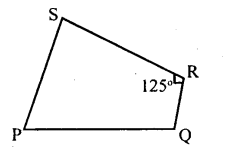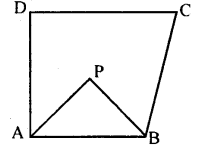# KSEEB Solutions for Class 8 Maths Chapter 15 Quadrilaterals Ex 15.1

Students can Download Maths Chapter 15 Quadrilaterals Ex 15.1 Questions and Answers, Notes Pdf, KSEEB Solutions for Class 8 Maths helps you to revise the complete Karnataka State Board Syllabus and score more marks in your examinations.

## Karnataka Board Class 8 Maths Chapter 15 Quadrilaterals Ex 15.1

Question 1.
Two angles of a quadrilateral are 70° and 130° and the other two angles are equal. Find the measure of these two angles.
Let the equal angles be x
70 + 130 + x + x = 360°
[Sum of the angles of a quadrilateral 360°]
200 + 2x = 360°
2x = 160
x =$\frac { 160 }{ 2 }$
x = 80°
Each of the other two angles is 80°

Question 2.
In the figure. Suppose ∠P and ∠Q are supplementary angles and ∠R = 125 °. Find the measure of∠S.∠P + ∠Q +∠R + ∠s = 360°
[Sum of the angles of a quadrilateral]
180 + 125° + ∠S = 360°
[∠P and ∠Q are supplementary]
305 + ∠S = 360°
∠ S = 360 – 305
∠S = 55°

Question 3.
Three angles of a quadrilateral are in the ratio 2:3:5 and the fourth angles are 90°. Find the measure of the other three angles.
Three angles are in the ratio 2 : 3 : 5 Let the angles be 2x, 3x and 5x
[Sum of the angles of a quadrilateral]
2x + 3x + 5x + 90° = 360°
10x + 90 = 360
10x = 360 – 90
10x = 270°
x =$\frac { 270 }{ 10 }$ = 27°
∴ 2x = 2 × 27 = 54°
3x = 3 × 27 = 81°
5x = 5 × 27 = 135°
Three angle are 54°, 81° & 135°

Question 4.
In the adjoining figure, ABCD is a quadrilateral such that ∠D + ∠C = 100°. The bisectors of ∠A and ∠B meet at P.
Determine ∠APB.∠A + ∠B + ∠C + ∠D = 360°
[Sum of the angles of a quadrilateral]
∠A + ∠B = 360 – 100
∠A + ∠B = 260$\frac { 1 }{ 2 }$ ∠A +$\frac { 1 }{ 2 }$  ∠B =$\frac { 1 }{ 2 }$ × 260°
[Multiplying by$\frac { 1 }{ 2 }$ ]
∠PAB + ∠PBA = 130°
[AP and BP are bisectors of ∠A and ∠B]
In ∆ APB. ∠APB +∠PAB +∠PB A = 180°
∠PAB + ∠PBA + ∠APB = 180°
[Sum of the angles of a triangle]
∠APB + 130 = 180°
∠APB = 180 – 130
∠ APB = 50°

error: Content is protected !!